Data tell a story. A purpose of data visualization is to convey that story to the reader in a clear and impactful way. Sometimes you can let the data "speak for themselves" in an unadorned graphic, but sometimes it is helpful to add reference lines to a graph to emphasize key features of the data.

This article discusses the REFLINE statement in PROC SGPLOT in SAS. This is a statement that I use daily. This article provides multiple "Getting Started" examples that show how to use the REFLINE statement to improve your graphs. Examples include:

• Display a reference line at a value such as a mean or median
• Add labels to a reference line
• Display normal ranges for measurements
• Use reference lines for a categorical variable on a discrete axis

### Basic reference lines

The REFLINE statement in PROC SGPLOT is easy to use. You can specify one or more values (separated by spaces) or you can specify a variable in the data set that contains the values at which to display the reference lines. You then use the AXIS=X or AXIS=Y option to specify which axis the reference lines are for. The reference lines are perpendicular to the axis.

A simple use of a reference line is to indicate a reference value on a histogram. For example, a healthy total cholesterol level is less than 200 mg/dL. A "borderline" (or moderately elevated) cholesterol level is between 200 and 240 mg/dL. A cholesterol level that is 240 or more is considered high. The Sashelp.Heart data set contains cholesterol and blood pressure information for patients in a heart study. The following histogram shows the distribution of cholesterol values for 5,195 subjects. You can use reference lines to indicate good, borderline, and high cholesterol.

```data Heart; set sashelp.Heart(where=(Cholesterol<400)); keep Cholesterol Systolic; run;   proc sgplot data=Heart; histogram Cholesterol; refline 200 240 / axis=x lineattrs=(thickness=3 color=darkred pattern=dash); /* Note: Order matters. Put REFLINE stmt first if you want it behind the bars */ run;```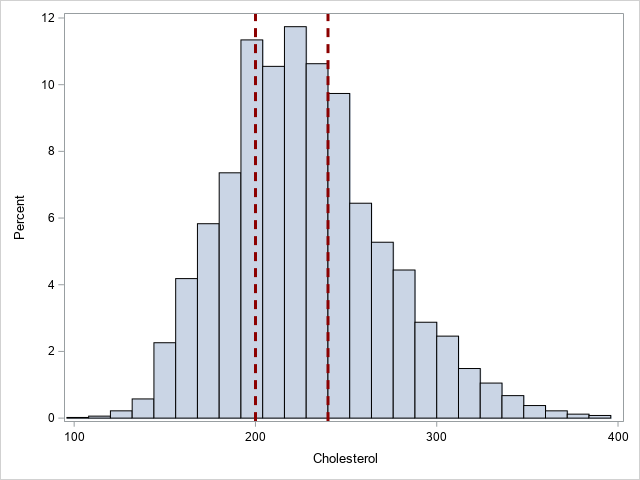In this example, I used the optional LINEATTRS= option to show how to change the color, line pattern, and thickness of the reference lines.

### Reference lines with labels

If you might want to add a label to the reference lines, you can use the LABEL= option to specify one or more labels. You can use the LABELLOC= option to put the label inside or outside the data area of the graph. I like "outside" (the default) because then the line does not interfere with the label. You can use the LABELPOS= option to specify whether the label is displayed at the top or bottom (for a vertical reference line) or at the left or right (for a horizontal reference line). The following example adds labels to the previous example.

```proc sgplot data=Heart; histogram Cholesterol; refline 200 240 / axis=x lineattrs=(thickness=3 color=darkred pattern=dash) label=("Borderline" "High"); /* OPT: labelloc=inside labelpos=max; */ run;```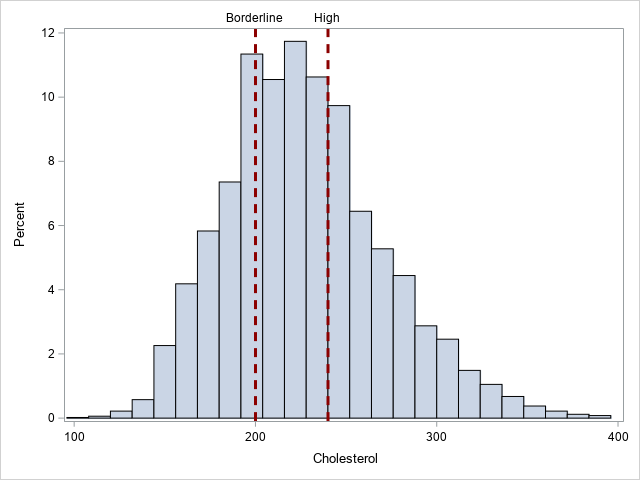### Reference lines at computed locations

Sometimes the reference values are the result of a computation. The REFLINE values and the LABEL= option can come from variables in a SAS data set. For multiple values, you probably want to arrange the values in "long form."

A good example is displaying descriptive statistics such as a mean, median, and percentiles. The following call to PROC MEANS computes three statistics for the Cholesterol variable: the median, the 25th percentile, and the 75th percentile. The output from PROC MEANS is one row and three columns, so I use PROC TRANSPOSE to convert the data set into long form, as follows:

```/* create a data set of statistics */ proc means data=Heart Median P25 P75; var Cholesterol; output out=MeansOut(drop=_TYPE_ _FREQ_) median=Median P25=P25 P75=P75; run;   proc transpose data=MeansOut Name=Stat out=Stats(rename=(Col1=CholValue)); run;   proc print data=Stats noobs; run;```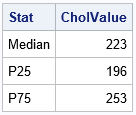You can append the statistics to the original data set and use PROC SGPLOT to create a histogram with reference lines that display the computed percentiles.

```data HeartChol; set Heart Stats; run;   proc sgplot data=HeartChol; histogram Cholesterol; refline CholValue / axis=x label=Stat lineattrs=GraphData2(thickness=3); run;```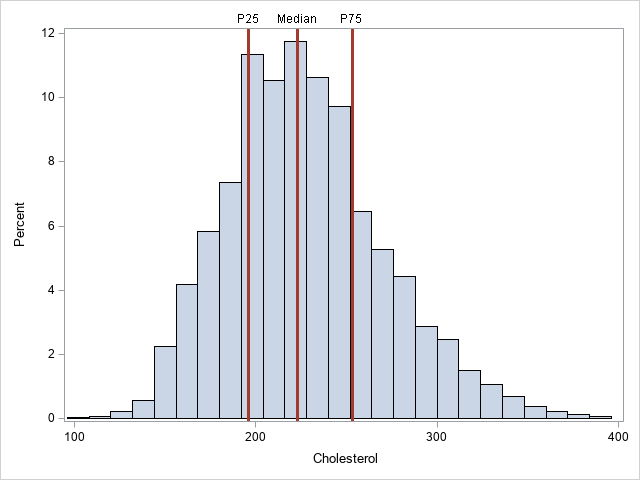In this example, I used the LINEATTRS=GRAPHDATA2 option to assign the style attributes of the lines. I used the THICKNESS= suboption to override the default thickness.

### Reference lines for 2-D plots

You can also add reference lines to one or both axes of a two-dimensional plot such as a scatter plot, heat map, or contour plot. The following graph shows a heat map of the cholesterol and systolic blood pressure values for more than 5,000 patients. The reference lines show clinical values for normal, slightly high, and high levels of both variables:

```title "Clinical Ranges of Systolic Blood Pressure and Cholesterol"; proc sgplot data=HeartStats; heatmap x=Cholesterol y=Systolic / colormodel=(CXDEEBF7 CX9ECAE1 CX3182BD ); refline 200 240 / axis=x label=('Borderline' 'High') lineattrs=GraphData2; refline 120 130 / axis=y label=('Elevated' 'Hypertensive') lineattrs=GraphData2; gradlegend / position=bottom; run;```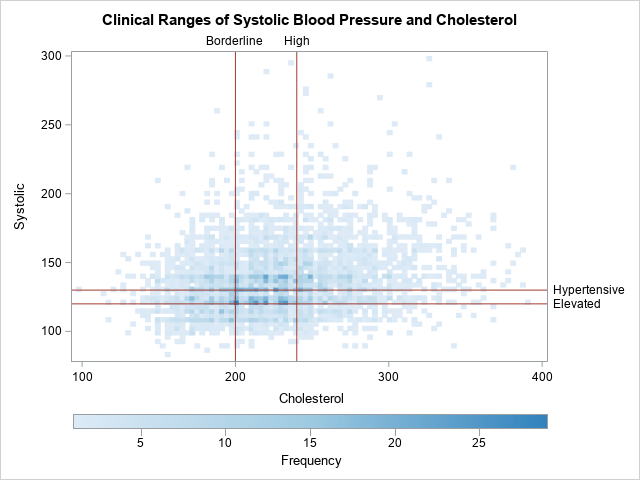### Reference lines for a discrete axis

You can also display reference lines on a discrete axis, although it is not common. One application that I can think of is displaying an expected value for a discrete probability distribution. Another application is simply drawing a line that separates one set of categories from another. In the following example, I use a reference line to indicate a fiscal year. Notice the following:

• If the categorical variable has a format, you need to specify the formatted value.
• By default, the reference line will be in the middle of the category. You can use the DISCRETEOFFSET= option and a value in the interval [-0.5, 0.5] to move the line left or right of center. Positive values move the line to the right; negative values move the line to the left. In the example, DISCRETEOFFSET=0.5 moves the line between the reference category and its neighbor to the right.
• The REFLINE statement supports a SPLITCHAR= option that you can use to split a long label across multiple lines.
```data Revenue; input Quarter Date9. Revenue; label Revenue = "Revenue (millions)"; format Quarter YYQ4.; datalines; 01Sep2018 1.5 01Dec2018 2.7 01Mar2019 1.2 01Jun2019 1.6 01Sep2019 1.4 01Dec2019 2.8 01Mar2020 0.8 ;   title "Quarterly Revenue for ABC Corp"; proc sgplot data=Revenue; vbar Quarter/ response=Revenue; /* for a discrete variable, specified the formatted value */ refline "19Q2" / axis=x discreteoffset=0.5 /* move ref line to right */ labelloc=inside label="Fiscal /Year " splitchar="/"; /* split label */ xaxis discreteorder=data; yaxis grid offsetmax=0.1; run;```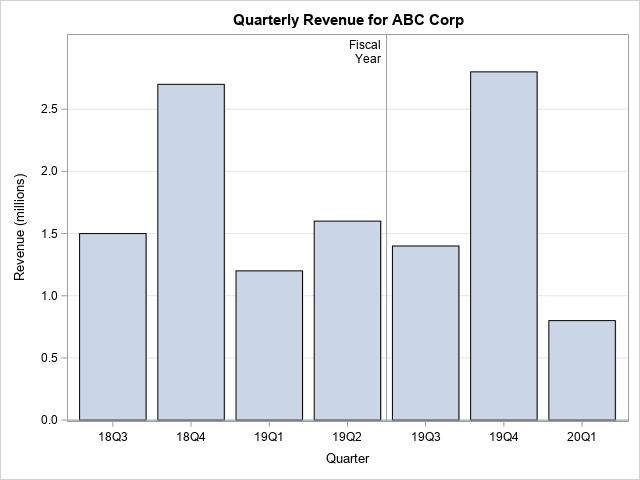### Getting fancy with reference lines

Because you can control the thickness of the reference lines, you can use them for many purposes. Sanjay Matange shows two creative uses for reference lines for a discrete axis:

### Summary

This article shows several ways to use the REFLINE statement in PROC SGPLOT to add information to your graphs. You can display a line to indicate a reference value or a sample statistic. You can display labels for reference lines. You can even use reference lines for a categorical variable on a discrete axis. Reference lines are a powerful way to enhance your graphs.

The post Add horizontal and vertical reference lines to SAS graphs: The REFLINE statement appeared first on The DO Loop.